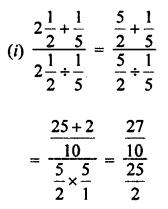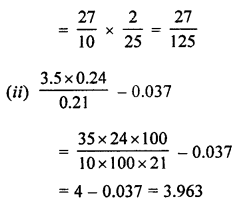## ML Aggarwal Class 7 Solutions for ICSE Maths Chapter 2 Fractions and Decimals Ex 2.7

Simplify the following (1 to 3):
Question 1.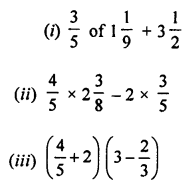Solution: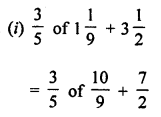Question 2.Solution: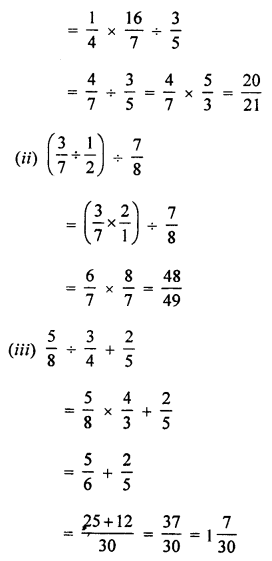Question 3.Solution: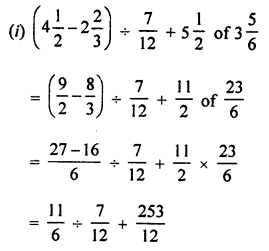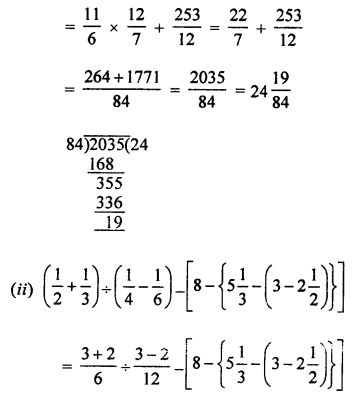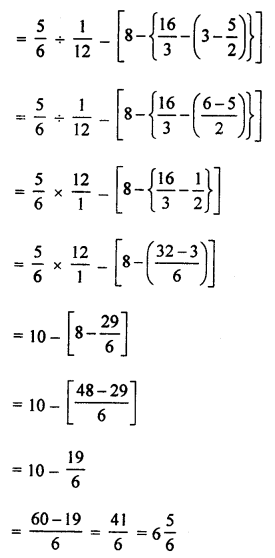Question 4.
Simplify the following:Solution:Question 5.
Simplify the following:Solution: